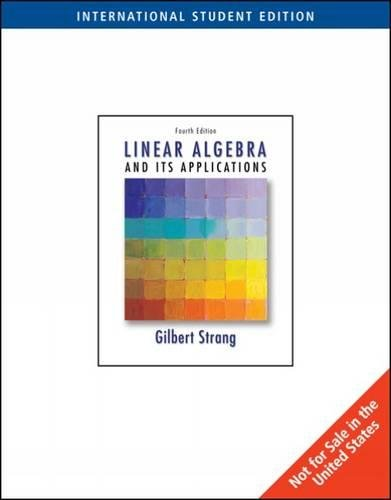• Free Shipping on all orders in Australia
• Over 7 million books in stock
• Proud to be B-Corp
• We aim to be carbon neutral by 2022
• Over 120,000 Trustpilot reviews
Item 1 of 0Linear Algebra and Its Applications by Strang Strang (Massachusetts Institute of Technology)

Condition - Like New
\$51.99
Out of stock

Summary

Demonstrates that linear algebra is a fascinating subject by showing both its beauty and value. Emphasizing on understanding, this book provides an introduction to vector spaces. The theory is motivated and reinforced by genuine applications, allowing pure mathematicians to teach applied mathematics.

Linear Algebra and Its Applications Summary

Linear Algebra and Its Applications by Strang Strang (Massachusetts Institute of Technology)

Renowned professor and author Gilbert Strang demonstrates that linear algebra is a fascinating subject by showing both its beauty and value. While the mathematics is there, the effort is not all concentrated on proofs. Strang's emphasis is on understanding. He explains concepts, rather than deduces. This book is written in an informal and personal style and teaches real mathematics. The gears change in Chapter 2 as students reach the introduction of vector spaces. Throughout the book, the theory is motivated and reinforced by genuine applications, allowing pure mathematicians to teach applied mathematics.

Linear Algebra and Its Applications Reviews

1. MATRICES AND GAUSSIAN ELIMINATION. Introduction. The Geometry of Linear Equations. An Example of Gaussian Elimination. Matrix Notation and Matrix Multiplication. Triangular Factors and Row Exchanges. Inverses and Transposes. Special Matrices and Applications. Review Exercises. 2. VECTOR SPACES. Vector Spaces and Subspaces. The Solution of m Equations in n Unknowns. Linear Independence, Basis, and Dimension. The Four Fundamental Subspaces. Networks and Incidence Matrices. Linear Transformations. Review Exercises. 3. ORTHOGONALITY. Perpendicular Vectors and Orthogonal Subspaces. Inner Products and Projections onto Lines. Least Squares Approximations. Orthogonal Bases, Orthogonal Matrices, and Gram-Schmidt Orthogonalization. The Fast Fourier Transform. Review and Preview. Review Exercises. 4. DETERMINANTS. Introduction. Properties of the Determinant. Formulas for the Determinant. Applications of Determinants. Review Exercises. 5. EIGENVALUES AND EIGENVECTORS. Introduction. Diagonalization of a Matrix. Difference Equations and the Powers Ak. Differential Equations and the Exponential eAt. Complex Matrices: Symmetric vs. Hermitian. Similarity Transformations. Review Exercises. 6. POSITIVE DEFINITE MATRICES. Minima, Maxima, and Saddle Points. Tests for Positive Definiteness. The Singular Value Decomposition. Minimum Principles. The Finite Element Method. 7. COMPUTATIONS WITH MATRICES. Introduction. The Norm and Condition Number. The Computation of Eigenvalues. Iterative Methods for Ax = b. 8. LINEAR PROGRAMMING AND GAME THEORY. Linear Inequalities. The Simplex Method. Primal and Dual Programs. Network Models. Game Theory. Appendix A: Computer Graphics. Appendix B: The Jordan Form. References. Solutions to Selected Exercises. Index.

1. MATRICES AND GAUSSIAN ELIMINATION. Introduction. The Geometry of Linear Equations. An Example of Gaussian Elimination. Matrix Notation and Matrix Multiplication. Triangular Factors and Row Exchanges. Inverses and Transposes. Special Matrices and Applications. Review Exercises. 2. VECTOR SPACES. Vector Spaces and Subspaces. The Solution of m Equations in n Unknowns. Linear Independence, Basis, and Dimension. The Four Fundamental Subspaces. Networks and Incidence Matrices. Linear Transformations. Review Exercises. 3. ORTHOGONALITY. Perpendicular Vectors and Orthogonal Subspaces. Inner Products and Projections onto Lines. Least Squares Approximations. Orthogonal Bases, Orthogonal Matrices, and Gram-Schmidt Orthogonalization. The Fast Fourier Transform. Review and Preview. Review Exercises. 4. DETERMINANTS. Introduction. Properties of the Determinant. Formulas for the Determinant. Applications of Determinants. Review Exercises. 5. EIGENVALUES AND EIGENVECTORS. Introduction. Diagonalization of a Matrix. Difference Equations and the Powers Ak. Differential Equations and the Exponential eAt. Complex Matrices: Symmetric vs. Hermitian. Similarity Transformations. Review Exercises. 6. POSITIVE DEFINITE MATRICES. Minima, Maxima, and Saddle Points. Tests for Positive Definiteness. The Singular Value Decomposition. Minimum Principles. The Finite Element Method. 7. COMPUTATIONS WITH MATRICES. Introduction. The Norm and Condition Number. The Computation of Eigenvalues. Iterative Methods for Ax = b. 8. LINEAR PROGRAMMING AND GAME THEORY. Linear Inequalities. The Simplex Method. Primal and Dual Programs. Network Models. Game Theory. Appendix A: Computer Graphics. Appendix B: The Jordan Form. References. Solutions to Selected Exercises. Index.

GOR010730358
9780534422004
0534422004
Linear Algebra and Its Applications by Strang Strang (Massachusetts Institute of Technology)
Used - Like New
Paperback
Cengage Learning, Inc
20060528
496
null null null null null null null null null null
Book picture is for illustrative purposes only, actual binding, cover or edition may vary.
The book has been read, but looks new. The book cover has no visible wear, and the dust jacket is included if applicable. No missing or damaged pages, no tears, possible very minimal creasing, no underlining or highlighting of text, and no writing in the margins